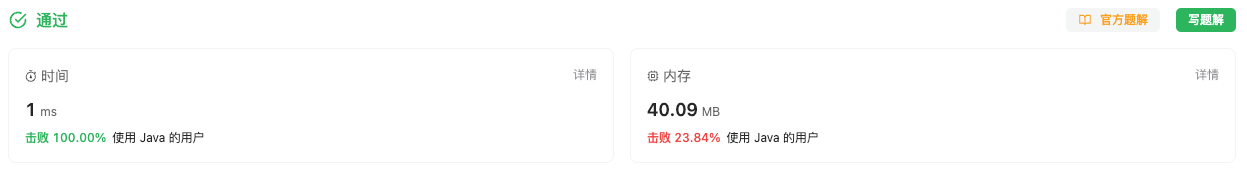#### 2609. 最长平衡子字符串

###### 来源: 每日一题 2023.11.08

``````输入：s = "01000111"

``````

``````输入：s = "00111"

``````

``````输入：s = "111"

``````

• `1 <= s.length <= 50`
• `'0' <= s[i] <= '1'`
``````class Solution {
public int findTheLongestBalancedSubstring(String s) {

}
}
``````

#### 分析与题解

• 计数模拟法

这个题其实我们只需要知道, 什么时候启动下一个子串什么时候比较最终结果就好.

为了满足题意, 停止条件只会有一个, 那就是前面有 n 个 `1` , 当前位置数字是 `0`, 就要停止了, 这时候判断子串的长度. 输出到最终结果中, 同时这时候也是新一轮的开始.

由于前面的 `1``0` 的个数不一样, 例如一个这样的数字 `001110`, 在最后一个 `0` 满足了条件停止了, 所以, 我们得到了 `00111` 那么平衡子字符串应该是 `0011`, 这时候我们只需要取前面子串的 `0``1` 数量较少的个数乘以2就是平衡子字符串的长度.

接下来, 我们看一下解题过程. 首页我们定义两个常量 `zero``one` 用来存储子串的 `0``1` 的数量情况.

``````int zero = 0, one = 0;
``````

然后, 我们进行遍历, 先判断当前是 `0` 的情况, 当 `one > 0` 也就是前面子串有 `1` 就进入停止逻辑. 停止逻辑中除了要输出maxLength, 还要清空 `zero``one`, 来为下一次子串判断做准备.

``````if (s.charAt(i) == '0') {
if (one != 0) {
// 有1 停止了
// 找两者中较小的值, 然后乘以2与最终结果做比较
int minCount = Math.min(zero, one);
maxLength = Math.max(maxLength, minCount * 2);
// 清空数据
zero = 0;
one = 0;
// 再进行下一轮
}
zero++;
}
``````

对于 当前下标对应数字是 `1` 的情况, 我们只需要判断前面有 `0`, 我们就对 `one` 进行 `+1` 操作.

``````if (zero > 0) {
one++;
}
``````

当然了, 在遍历完成之后, 最后一个子串肯定没法在循环中判断, 我们直接在外部再进行一次判断即可.

``````// 遍历完成,判断最后的一组数据情况
if (zero > 0 && one > 0) {
int minCount = Math.min(zero, one);
maxLength = Math.max(maxLength, minCount * 2);
}
``````

那么接下来, 我们就看一下整体的题解过程.

``````class Solution {
public int findTheLongestBalancedSubstring(String s) {
int zero = 0, one = 0;
int maxLength = 0;
for(int i = 0; i < s.length(); i++) {
if (s.charAt(i) == '0') {
if (one != 0) {
// 有1 停止了
// 找两者中较小的值, 然后乘以2与最终结果做比较
int minCount = Math.min(zero, one);
maxLength = Math.max(maxLength, minCount * 2);
// 清空数据
zero = 0;
one = 0;
// 再进行下一轮
}
zero++;
} else {
if (zero > 0) {
one++;
}
}
}
// 遍历完成,判断最后的一组数据情况
if (zero > 0 && one > 0) {
int minCount = Math.min(zero, one);
maxLength = Math.max(maxLength, minCount * 2);
}
return maxLength;
}
}
``````

复杂度分析:

• 时间复杂度: O(n), 与字符串数组长度相关的时间复杂度.
• 空间复杂度: O(1), 常量基本的时间复杂度.

结果如下所示.IT界无底坑洞栋主 欢迎加Q骚扰:676758285